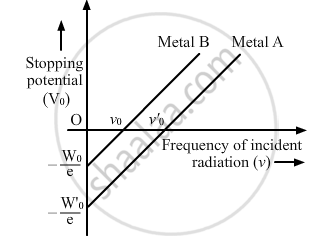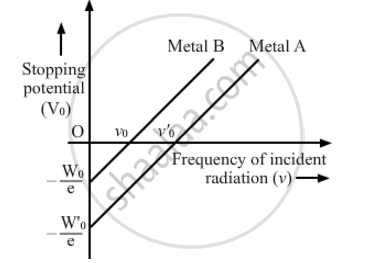# Which One of the The Variation of Stopping Potential with Frequency of Incident Radiation for Two Photosensitive Metals a and B Has Higher Value of Work-function? - Physics

The graph shows the variation of stopping potential with frequency of incident radiation for two photosensitive metals A and B. Which one of the two has higher value of work-function? Justify your answer.The graph shows variation of stopping potential V0 versus frequency of incident radiation v for two photosensitive metals A and B. Which of the two metals has higher threshold frequency and why?#### Solution

eV0=hνhν0

V_0-h/ev-h/ev_0

Here, e is the charge of an electron, Vo is the stopping potential, h is Planck's constant, ν0 is the threshold frequency and ν is the frequency of the incident light.

On comparing with y=mx+c, we find that the more the magnitude of intercept on y or V0 axis, the more is the threshold frequency (ν0).
From the graph, the threshold frequency for metal A is greater than that for metal B. Hence, the work function for metal A is greater than that for metal B.

Concept: Photoelectric Effect - Hertz’s Observations
Is there an error in this question or solution?
2013-2014 (March) All India Set 2

Share